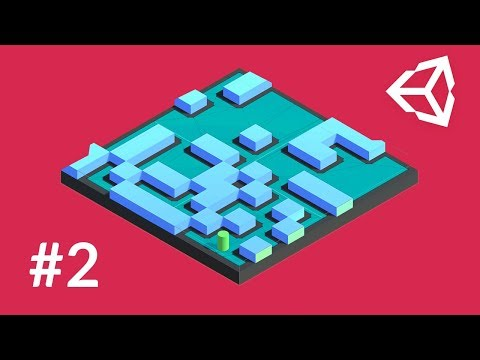Making it Dynamic

Сложность: Базовая

Learn how to update your NavMesh at runtime! This video was produced by Brackeys.ObstacleAnimation

Code snippet

using System.Collections;
using System.Collections.Generic;
using UnityEngine;

public class ObstacleAnimation : MonoBehaviour {

public float speed = .2f;
public float strength = 9f;

private float randomOffset;

// Use this for initialization
void Start () {
randomOffset = Random.Range(0f, 2f);
}

// Update is called once per frame
void Update () {
Vector3 pos = transform.position;
pos.x = Mathf.Sin(Time.time * speed + randomOffset) * strength;
transform.position = pos;
}
}

LevelGenerator

Code snippet

using UnityEngine;

public class LevelGenerator : MonoBehaviour {

public NavMeshSurface surface;
public int width = 10;
public int height = 10;

public GameObject wall;
public GameObject player;

private bool playerSpawned = false;

// Use this for initialization
void Start () {
GenerateLevel();
surface.BuildNavMesh();
}

// Create a grid based level
void GenerateLevel()
{
// Loop over the grid
for (int x = 0; x <= width; x+=2)
{
for (int y = 0; y <= height; y+=2)
{
// Should we place a wall?
if (Random.value > .7f)
{
// Spawn a wall
Vector3 pos = new Vector3(x - width / 2f, 1f, y - height / 2f);
Instantiate(wall, pos, Quaternion.identity, transform);
} else if (!playerSpawned) // Should we spawn a player?
{
// Spawn the player
Vector3 pos = new Vector3(x - width / 2f, 1.25f, y - height / 2f);
Instantiate(player, pos, Quaternion.identity);
playerSpawned = true;
}
}
}
}
}